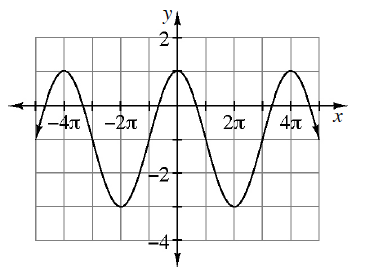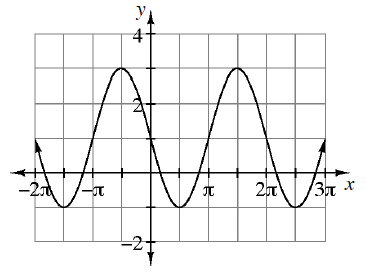### Home > PC3 > Chapter 4 > Lesson 4.1.3 > Problem4-48

4-48.

Write an equation for each graph below.

1.This equation is most easily written as a cosine function:
1. Where is the midline?
2. What is the amplitude?
3. Identify the period and calculate "$b$".

Substitute the information in the general equation for cosine.

1.This equation is most easily written as a negative sine function:
1. Where is the midline?
2. What is the amplitude?
3. Identify the period and calculate "$b$".

Substitute the information in the general equation for sine.